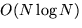Next: Hierarchical Collision Detection Methods Up: Introduction to Advanced Computer Previous: Introduction

# Sweep and Prune

Given a number N of objects, O(N2) object pairs have to be checked for collision. In general, the objects in most of the pairs aren't even close to each other so we should be able to eliminate them quickly. To do this we use a technique called Sweep and Prune ([CLMP95]). In this section I will briefly introduce this technique.

To determine whether two objects are close enough to potentially collide, the Sweep and Prune checks whether the axis aligned bounding boxes of the respective objects overlap. If they do, further investigation is necessary. If not, the objects can't possibly collide and the algorithm can move on. To determine whether two bounding boxes overlap, the algorithm reduces the 3D problem to three simpler 1D problems. It does so by determining the intervals occupied by the bounding volume along each of the x,y and z axes. If and only if the intervals of two bounding volumes overlap in all of the three dimensions, the objects corresponding to these bounding volumes must overlap. To determine which intervals of the objects along an axis overlap, the list of the intervals is sorted. Normally, using quick-sort, this would be anprocess. However, by exploiting frame coherence (the similarity between situations in two subsequent frames) we can sort the lists in an expected (O(N), using insertion sort.

Another difficult part in the Sweep and Prune approach is the maintenance of the bounding volume. If the objects in the scene move or rotate, the previously calculated bounding boxes are invalid. It is important to be able to update the boxes as quickly as possible. Again, we can do this by exploiting frame coherence.

The algorithm's performance is of course dependent on the application and the typical situations that occur in that application. Many variations exists, such as reducing the overlap problem by only 1 dimension and using a rectangle intersection test. It is also possible to choose other types of bounding volumes that might be faster to update but produce a less accurate approximation of the object.Next: Hierarchical Collision Detection Methods Up: Introduction to Advanced Computer Previous: Introduction
Marc Ramaekers
5/17/1999